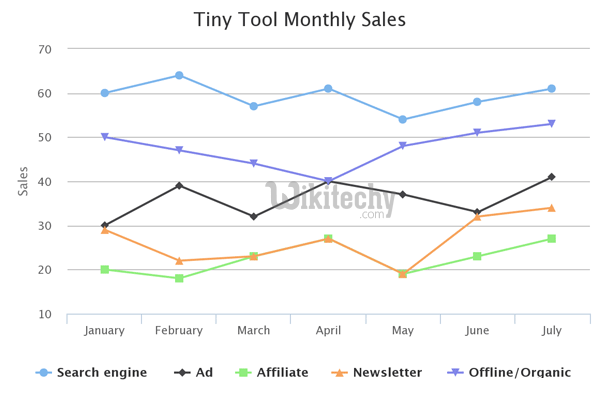# Google Chart - google chart tutorial - Basic Line Chart With visible points - chart js - google graphs - google charts examples

## What is Visible Points?

• This corresponds to the requirement that , where denotes the greatest common divisor.
• The plots above show the first few points visible from the origin.
• An invisible figure is a polygon all of whose vertices (with possibly degenerate edges when restricted on a grid) are invisible from the origin.## Configuration

• The code which is given below shows us the configuration for basic line chart with visible points and we have added pointVisible configuration to show data points

## Code:

• The program which is given below shows us the full program of basic line chart with visible points which is given below: